CBSE Class 12 Sample Paper for 2022 Boards (MCQ Based - for Term 1)

Class 12
Solutions of Sample Papers and Past Year Papers - for Class 12 Boards

## (a) 8  (b) 10 (c) 4  (d) −8

This question is inspired from Example 5 - Chapter 3 Class 12   - Matrices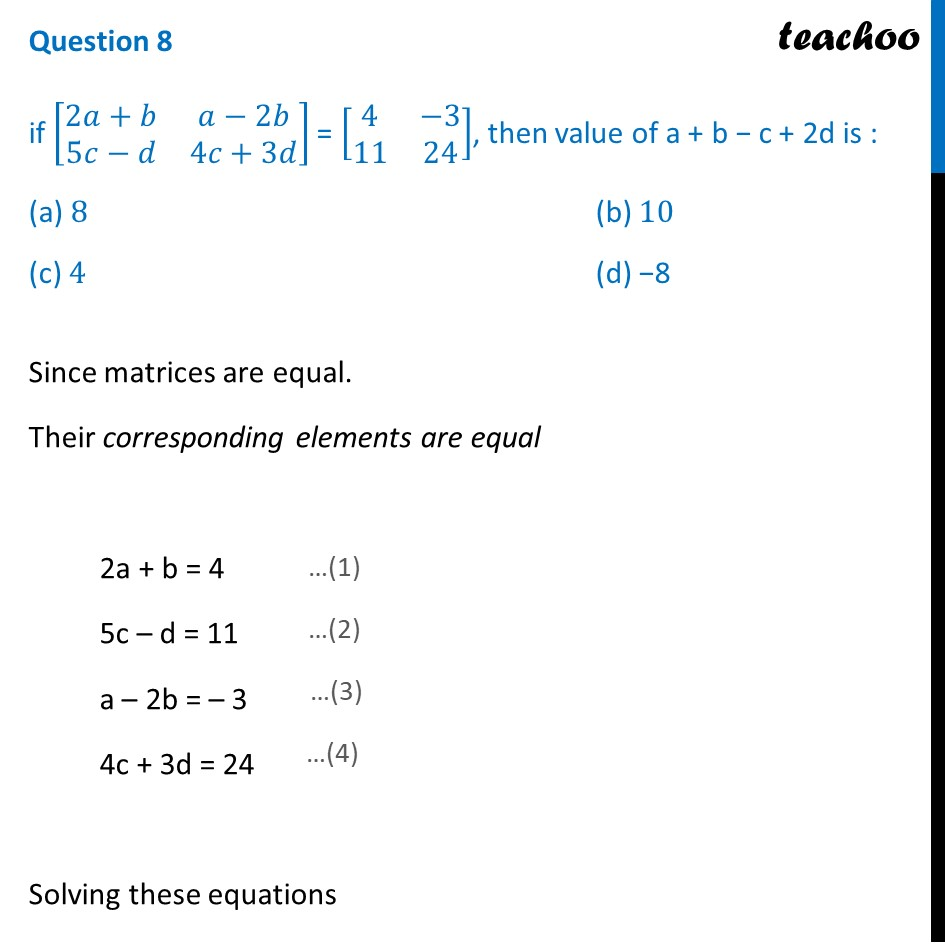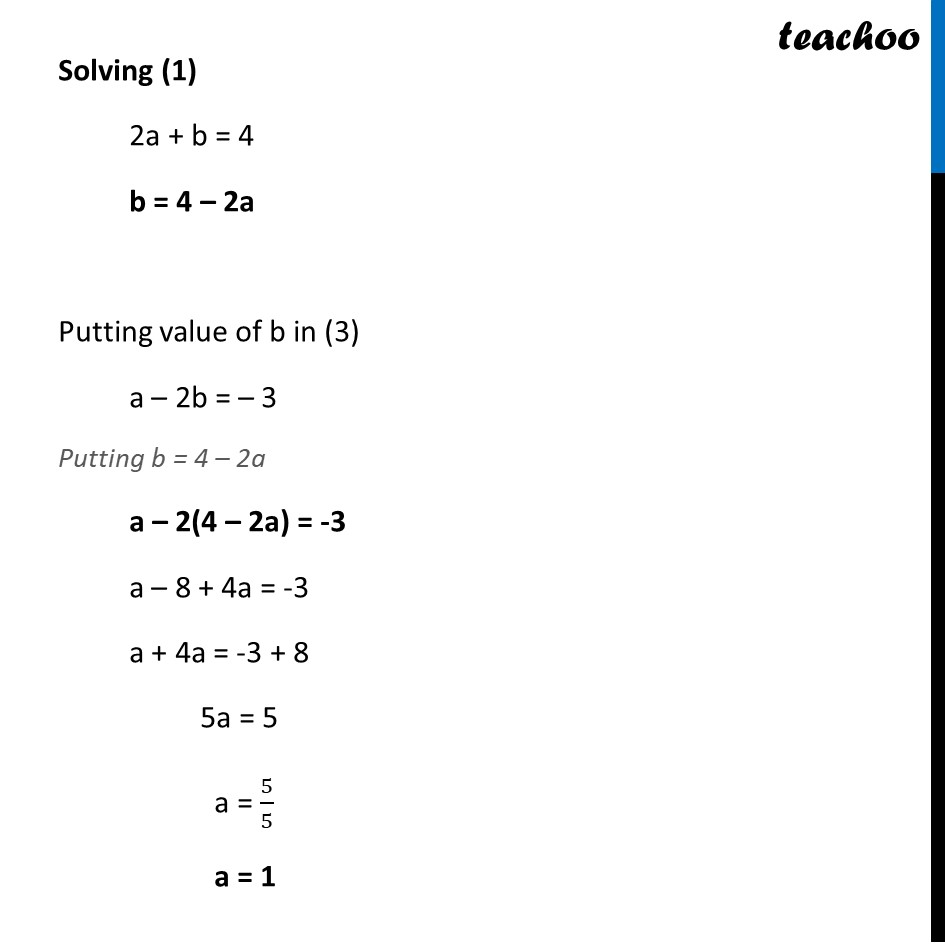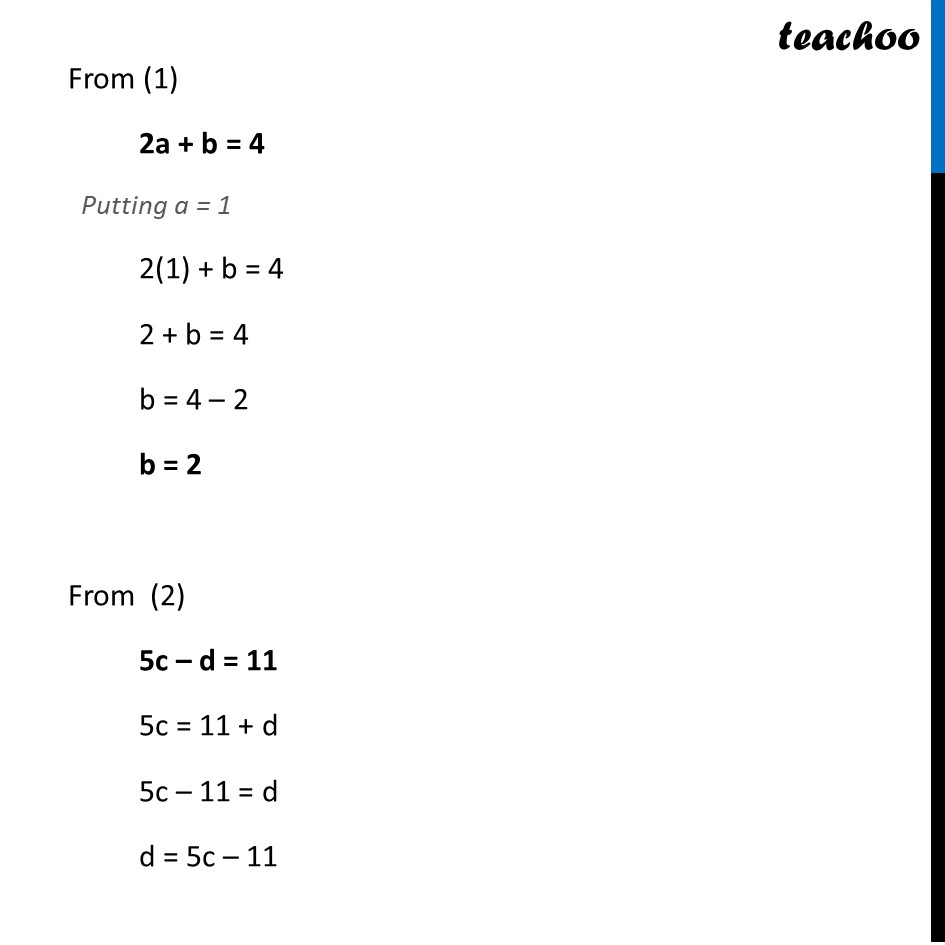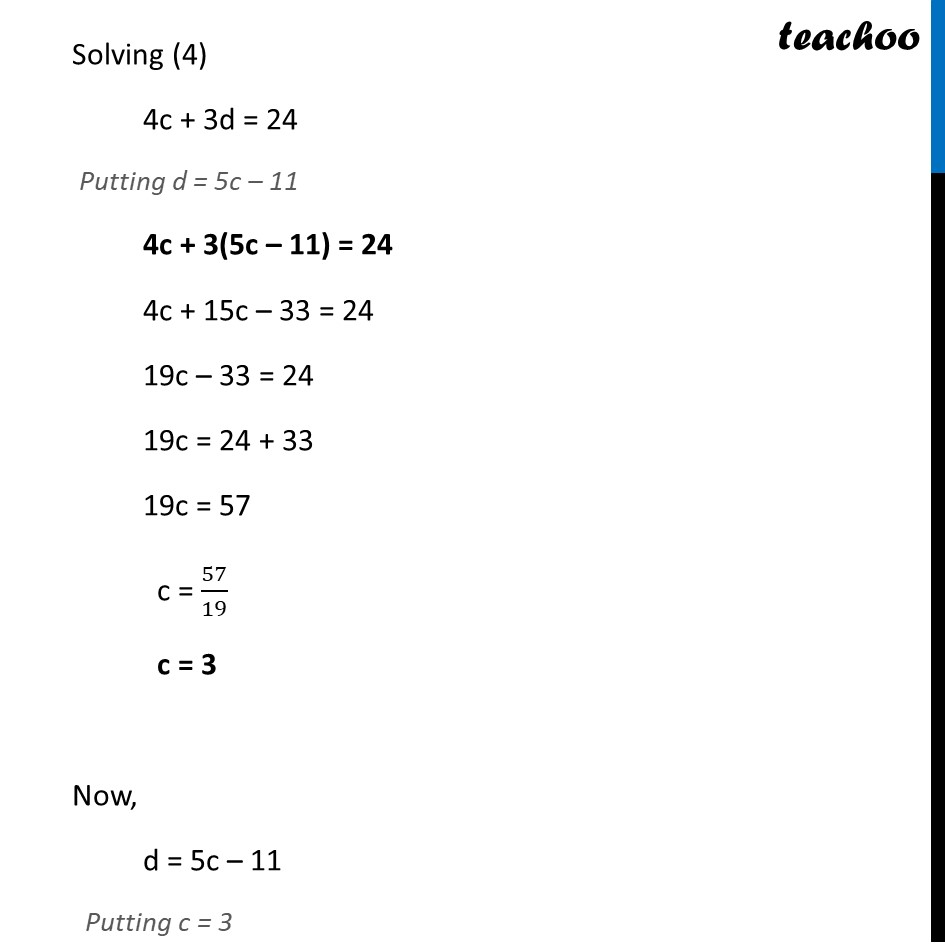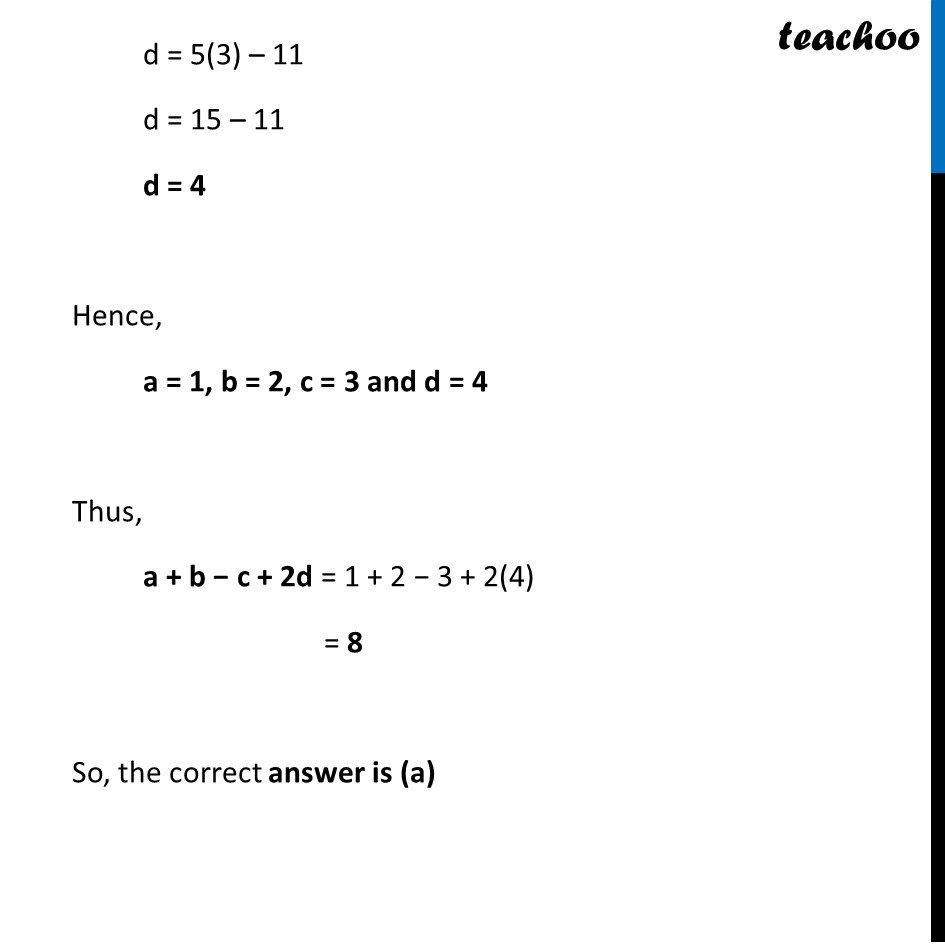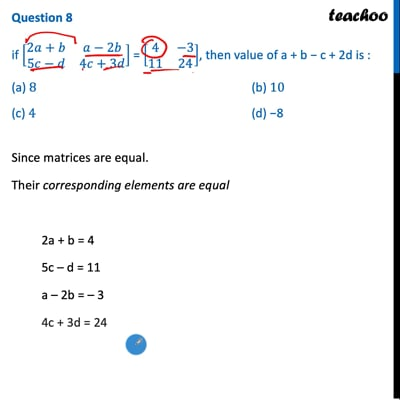This video is only available for Teachoo black users

Introducing your new favourite teacher - Teachoo Black, at only ₹83 per month

### Transcript

Question 8 if [■8(2𝑎+𝑏&𝑎−2𝑏@5𝑐−𝑑&4𝑐+3𝑑)] = [■8(4&−3@11&24)], then value of a + b − c + 2d is : (a) 8 (b) 10 (c) 4 (d) −8 Since matrices are equal. Their corresponding elements are equal 2a + b = 4 5c – d = 11 a – 2b = – 3 4c + 3d = 24 Solving these equations Since matrices are equal. Their corresponding elements are equal 2a + b = 4 5c – d = 11 a – 2b = – 3 4c + 3d = 24 Solving these equations Solving (1) 2a + b = 4 b = 4 – 2a Putting value of b in (3) a – 2b = – 3 Putting b = 4 – 2a a – 2(4 – 2a) = -3 a – 8 + 4a = -3 a + 4a = -3 + 8 5a = 5 a = 5/5 a = 1 From (1) 2a + b = 4 Putting a = 1 2(1) + b = 4 2 + b = 4 b = 4 – 2 b = 2 From (2) 5c – d = 11 5c = 11 + d 5c – 11 = d d = 5c – 11 Solving (4) 4c + 3d = 24 Putting d = 5c – 11 4c + 3(5c – 11) = 24 4c + 15c – 33 = 24 19c – 33 = 24 19c = 24 + 33 19c = 57 c = 57/19 c = 3 Now, d = 5c – 11 Putting c = 3 d = 5(3) – 11 d = 15 – 11 d = 4 Hence, a = 1, b = 2, c = 3 and d = 4 Thus, a + b − c + 2d = 1 + 2 − 3 + 2(4) = 8 So, the correct answer is (a)